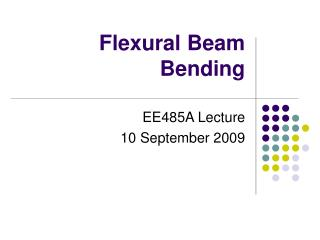# Flexural Beam Bending - PowerPoint PPT PresentationDownload PresentationFlexural Beam Bending

Flexural Beam Bending
Download Presentation## Flexural Beam Bending

- - - - - - - - - - - - - - - - - - - - - - - - - - - E N D - - - - - - - - - - - - - - - - - - - - - - - - - - -
##### Presentation Transcript

1. Flexural Beam Bending EE485A Lecture 10 September 2009

2. Mass-Spring Systems k = 1 N/m k = 1 N/m k = 1 N/m k = 1 N/m 1 kg 1 kg k = 1 N/m 1 kg Given that gravity is acting on these masses, how far will each of these masses deflect?

3. Springs are everywhere • Mass-spring systems ubiquitous in MEMS • Goal for today: to derive the spring constant for a MEMS beam from its geometry and material properties.

4. Beam Boundary Conditions • Beams are classified according to their supports

5. Identifying Boundary Conditions Fixed-fixed bridges (b,c*,f,h*) Fixed-free cantilevers (a,d,e,g,i,j) Four fixed-guided beams connect to rigid shuttle (k)

6. Pure Bending • Defined as when the moment is constant throughout the beam • Upper part of the beam is shortened and is in compression • Lower part of the beam is lengthened and is in tension • Neutral plane is dividing line, defined as surface with no longitudinal change in length.

7. y F Strain-Curvature Relation dq x y=0 The strain and stress vary through the thickness of the beam– they are greatest at its farthest distance from the neutral axis! Length of segment before bending: dx Length of segment after bending: dx - yk Strain curvature relation: sx = -ky

8. Normal stresses in beams y Elemental bending moment: sx Integrating to find total moment M x Leads to Beam Equation:

9. Calculating the moment of inertia Really the “second moment or inertia” or “second moment of area” For applying these equations, y is in the direction that the beam is bending. t w Prove:

10. Orientation matters • Determine the proper moments of inertia to use for the case of force F1 and force F2 F1 1 m 10 m F2

11. Deflection of Beams F x y New definition to y and x, treating beam as thin sheet at neutral axis plane

12. F Example Prove that deflection, y, at point x is given by: Prove that the tip deflection, ymax, is given by:

13. F Finding spring constants • Use the relation between the Force and tip displacement to determine the effective spring constant for the beam. Effective Spring Constant, k

14. A Practical Application A simplified silicon accelerometer is shown below, which is designed to detect acceleration in the –y direction. For silicon, E = 130 GPa, density = 2300 kg/m3 Force direction t = 1 mm W = 10 mm H = 10 mm L = 200 mm • Determine the spring constant of the beam. • Determine how far the tip mass will move if the sensor encounters an acceleration of 100g. • How could you make this device more sensitive? (More deflection for the same acceleration)